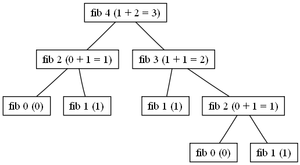# Hylomorphism (computer science)

﻿
Hylomorphism (computer science)

In computer science, and in particular functional programming, a hylomorphism is a recursive function, corresponding to the composition of an anamorphism (which first builds a set of results; also known as 'unfolding') and a catamorphism (which then folds these results into a final return value). Fusion of these two recursive computations into a single recursive pattern then avoids building the intermediate data structure. This is a particular form of the optimizing program transformation techniques collectively known as deforestation. The categorical dual of a hylomorphism is called a metamorphism, and is a catamorphism followed by an anamorphism.

## Formal definition

A hylomorphism$h : A \rightarrow C$ can be defined in terms of its separate anamorphic and catamorphic parts.

The anamorphic part can be defined in terms of a unary function$g : A \rightarrow B \times A$ defining the list of elements in B by repeated application ("unfolding"), and a predicate$p : A \rightarrow \text{Boolean}$ providing the terminating condition.

The catamorphic part can be defined as a combination of an initial value$c \in C$ for the fold and a binary operator$\oplus : B \times C \rightarrow C$ used to perform the fold.

Thus a hylomorphism$h\,a = \begin{cases} c & \mbox{if } p\,a \\b \oplus ha' & \mbox{otherwise} \end{cases}$

(where (b,a') = ga) may be defined (assuming appropriate definitions of p, g, h).

### Notation

An abbreviated notation for the above hylomorphism is$h = [\![(c, \oplus),(g, p)]\!]$.

## Hylomorphisms in practice

### Lists

Lists are common data structures, as they naturally reflect linear computational processes arising in repeated (iterative) chain of applications of a function. As such, it is sometimes necessary (or, at least, preferable) to generate a temporary list of intermediate results before performing an operation to reduce this list to a single final results.

One example of a commonly encountered hylomorphism is the canonical factorial function.

factorial :: Integer -> Integer
factorial n
| n == 0 = 1
| n > 0 = n * factorial (n - 1)


In the previous example (written in Haskell, a purely functional programming language) it can be seen that this function, applied to any given valid input, will generate a linear call tree isomorphic to a list. For example, given n = 5 it will produce the following:

factorial 5 = 5 * (factorial 4) = 120
factorial 4 = 4 * (factorial 3) = 24
factorial 3 = 3 * (factorial 2) = 6
factorial 2 = 2 * (factorial 1) = 2
factorial 1 = 1 * (factorial 0) = 1
factorial 0 = 1


In this example, the anamorphic part of the process is the generation of the call tree which is isomorphic to the list [1, 1, 2, 3, 4, 5]. The catamorphism, then, is the calculation of the product of the elements of this list. Thus, in the notation given above, the factorial function may be written$\text{factorial} = [\![(1,\times),(g, p)]\!]$ where$g\ n = (n, n - 1)$ and$p\ n = (n = 0)$.

### Trees

However, the term 'hylomorphism' does not apply solely to functions acting upon isomorphisms of lists. For example, a hylomorphism may also be defined by generating a non-linear call tree which is then collapsed. An example of such a function is the function to generate the nth term of the Fibonacci sequence.

 fibonacci :: Integer -> Integer
fibonacci n
| n == 0 = 0
| n == 1 = 1
| n > 1 = fibonacci (n - 2) + fibonacci (n - 1)Call tree for fibonacci 4.

This function, again applied to any valid input, will generate a call tree which is non-linear. In the example on the right, the call tree generated by applying the fibonacci function to the input 4.

This time, the anamorphism is the generation of the call tree isomorphic to the tree with leaf nodes 0, 1, 1, 0, 1 and the catamorphism the summation of these leaf nodes.

Wikimedia Foundation. 2010.

### Look at other dictionaries:

• Deforestation (computer science) — In the theory of programming languages in computer science, deforestation (also known as fusion) is a program transformation to eliminate tree structures. The term deforestation was originally coined by Philip Wadler in his paper Deforestation:… …   Wikipedia